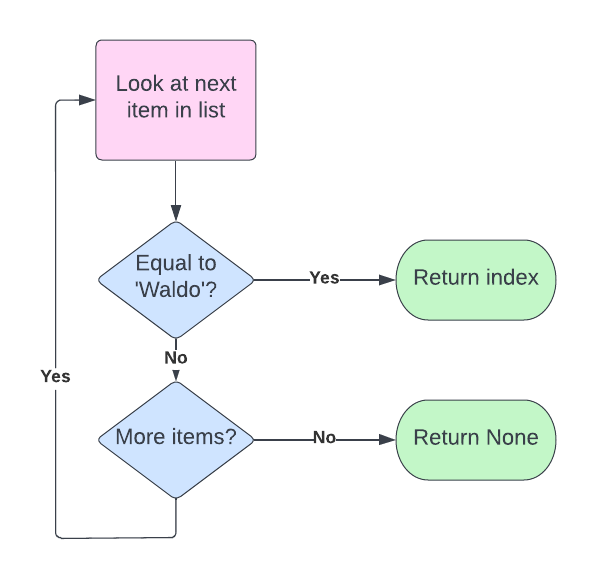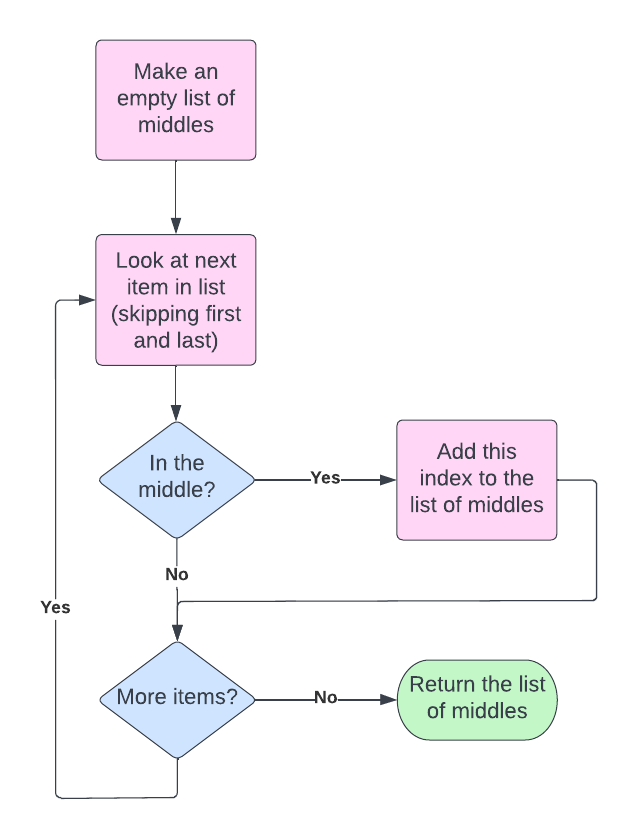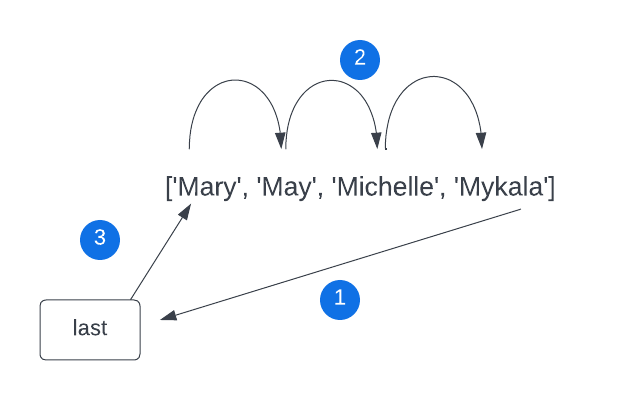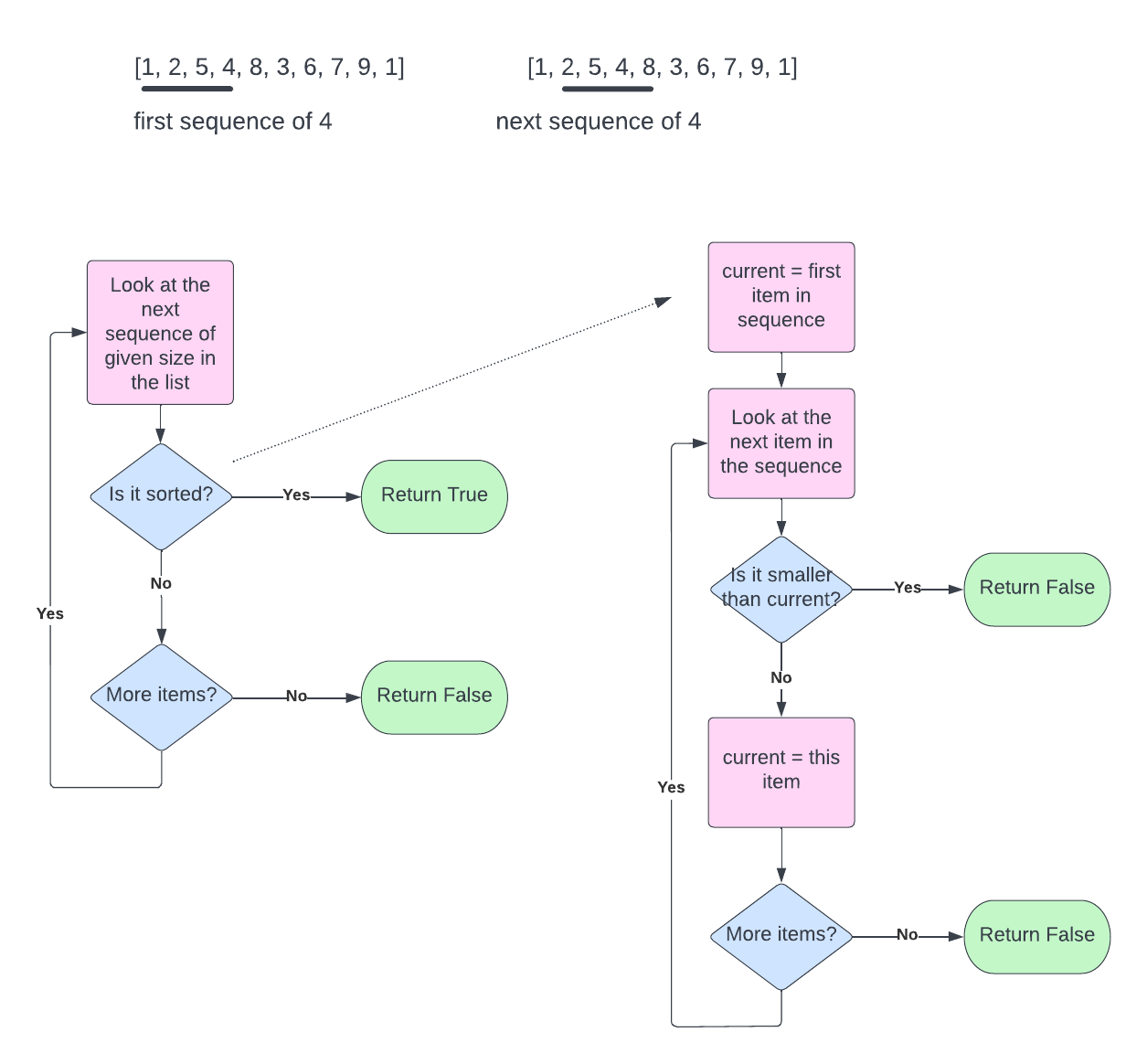Computer Science

# Practice with indexing

Let’s write some functions that practice what we’ve learned with indexing into lists.

## Where’s Waldo?

You have a list of names. Write a function that returns the index of ‘Waldo’. Return None if Waldo is not present.

def wheres_waldo(names):
pass

wheres_waldo(['Juan','William','Wally','Waldo','Wilford','Winston'])

Here is a flow chart for this algorithm:Putting this into words:

• for each string in the list
• check if the string is equal to ‘waldo’
• if it is, return the index
• return None by default

Here is now that translates into code:

def wheres_waldo(names):
for index in range(len(names)):
if names[index] == 'Waldo':
return index
return None

## Middles

Find all positions in a list where the value is greater than the value on the left and less than the value on the right.

The first and last positions do not have neighbors on both sides and should not be part of the output.

def find_middle_positions(items):
return []

numbers = [1, 5, 3, 7, 8, 2, 3, 4, 6, 9, 0, 2, 7]
find_middle_positions(numbers)

Try drawing a flow chart on your own.

Here is a flow chart:And here is this algorithm in English:

• make an empty list of middles

• for each item in the list (except first and last)

• is it in the middle?
• add it to the list of middles
• return the list of middles

Finally, here is some code that implements this algorithm:

def find_middle_positions(items):
middles = []
for index in range(1, len(items) - 1):
if items[index] > items[index - 1] and items[index] < items[index + 1]:
middles.append(index)
return middles

## Roll over

Given a list, shift every item to the left. The right-most item becomes None. Return the left-most item.

For example:

roll_over(['Mary', 'May', 'Michelle', 'Mykala'])

This should return Mary and the list should now contain ['May', 'Michelle', 'Mykala', None].

It helps to draw this out:In step (1) we save the first item into a variable called first. In step (2) we shift items to the left. In step (3) we put None into the last spot. At the end, we can return first.

Here is how we can translate that into code:

def roll_over(items):
# keep track of first item
first = items

# Shift everyone else left
for index in range(len(items)-1):
items[index] = items[index + 1]

# Put a None at the end
items[-1] = None

return first

Notice that for the range we count from 0 to len(items) - 1. This is because we need item 1 to move into position 0, item 2 into position 1 and so forth. If we have a list of length 4, and we try to move item 4 into position 3 — there is no item 4 and this will cause an error! You can see how this follows from the diagram above.

Now if we call this code:

names = ['Mary', 'May', 'Michelle', 'Mykala']
print(roll_over(names))
print(names)

We will get:

Mary
['May', 'Michelle', 'Mykala', None]

## Rotate right

Given a list, shift every item to the right one space. Items on the end wrap around to the front.

This is similar to the above problem. Let’s draw it out:In step (1) we save the last item into a variable called last. In step (2) we shift items to the right. In step (3) we put last into the first spot. We don’t need to return anything.

Here is this algorithm in code:

def rotate_right(items):
"""Shift the items one space to the right. Wrap the item on the end around to the front.
Modifies the input list.
"""
last = items[-1]
for index in range(1, len(items)):
destination = len(items) - index
items[destination] = items[destination - 1]
items = last

Notice that we need to be careful about how we shift items. If we shift the first item into the second spot, then the second spot is overwritten before it is moved! So we have to move the second-to-last item into the last spot, and then the third-to-last item into the second-to-last-item, and so forth.

We do this by creating a variable called destination that is set to len(items) - index. This will make the destination the last item the first time through the loop, then the second-to-last item, etc. We move whatever is in destination - 1 into destination.

We can call this code as follows:

pets = ['fish', 'dog', 'cat', 'gerbil', 'gecko', 'salamander', 'parakeet']
rotate_right(pets)
print(pets)

and this will print:

['parakeet', 'fish', 'dog', 'cat', 'gerbil', 'gecko', 'salamander']

## Sorted sequence

A sorted sequence is a series of numbers in consecutive positions in a list that are in order. For example, this is a sorted sequence:

1, 5, 8

We want to write a function tells us whether a list of numbers contains a sorted sequence of a given size. For example,

numbers = [1, 2, 5, 4, 8, 3, 6, 7, 9, 1]
sorted_sequence(numbers, 4)

This should return True because we can find [3, 6, 7, 9] — a sequence of 4 numbers that is sorted.

This function takes two parameters:

def has_sorted_sequence(items, size):
pass

Here is a flow chart for this problem:The top part shows that we need to go through a list of numbers and look at every sequence of the requested size. Here we show what you would do if size is 4.

The left side shows a flow chart for the overall problem. We go through each sequence of the requested size, and check if it is sorted. If we find a sorted sequence, we immediately return True. Otherwise, we keep looping.

The right side shows a flow chart for checking if a sequence is sorted. We set current equal to the first item. Then we loop through all of the rest of the items. If we find an item that is smaller than current, we return False. Otherwise we set current equal to the current item. By default we return True.

We can write a separate function for each side of the flow chart.

### Code for the left side

Here is code for the left side:

def has_sorted_sequence(items, size):
"""Returns True if the list contains a sorted sequence of length size."""
for index in range(len(items) + 1 - size):
if is_sorted_sequence(items, index, size):
return True
return False

Our range goes from 0 to len(items) + 1 - size. Look at the example at the top of the flow chart. We have 10 items and we want to check if there is a sorted sequence of length 4. Then we need to check the first 4 items, the next four items … and we have to do this 7 times. The number of times we need to loop is equal to the length of the list (10), plus 1, minus the size (4) — 10 + 1 - 4 = 7.

### Code for the right side

Here is the code for the right side:

def is_sorted_sequence(items, index, size):
"""Returns True if the list contains a sorted sequence of length size starting at position index."""
for i in range(index + 1, index + size):
if items[i] < items[i-1]:
return False
return True

We are given a list of items, an index, and a size. Imagine that we have this parameters:

itemsindexsize
[1, 2, 5, 4, 8, 3, 6, 7, 9, 1]14

By using range(index + 1, index + size)`, we are looping from the 2nd number in this sequence to the last. We then check if any item in the sequence is less than the item before it.

iitems[i]items[i - 1]Less?
252No
345Yes

So we return False.

Eventually we call this function with:

itemsindexsize
[1, 2, 5, 4, 8, 3, 6, 7, 9, 1]54

And we have:

iitems[i]items[i - 1]Less?
663No
776No
897No

So we return True — we found a sorted sequence.